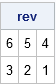A SAS user needed to convert a program from MATLAB into the SAS/IML matrix language and asked whether there is a SAS/IML equivalent to the fliplr and flipud functions in MATLAB. These functions flip the columns or rows (respectively) of a matrix; "LR" stands for "left-right" and "UD" stands for "up-down."

No, SAS/IML does not have equivalent built-in functions, but as Devo once sang: "When a problem comes along, you must whip it." This article shows how to implement these functions in SAS/IML. Or, to paraphrase Devo's maxim: "When a matrix comes along, you must flip it. It's not too late to flip it. Flip it good!"

### The FLIPLR and FLIPUD functions in SAS/IML

The key to reversing the columns or rows of a matrix is to use subscript notation. Recall that in the SAS/IML language, the expression A[ ,1] extracts the first column of A. (When the row subscript is not specified, it means "use all rows.") Similarly, A[ ,1:3] extracts the first three columns of A (in order), whereas A[ ,3:1] extracts the first three columns of A in reverse order. A similar notation is valid for rows. The expression A[3:1, ] extracts the first three rows of A in reverse order. Therefore, the following SAS/IML functions implement the functionality of the fliplr and flipud functions for matrices:

```proc iml; /* reverse columns of a matrix */ start fliplr(A); return A[ , ncol(A):1]; finish;   /* reverse rows of a matrix */ start flipud(A); return A[nrow(A):1, ]; finish;   /* test the functions on a 2x3 matrix */ x = {1 2 3, 4 5 6}; lr = fliplr(x); ud = flipud(x); print x, lr, ud;```### Reversing all elements

You can also reverse all elements of a matrix. Recall that a SAS/IML matrix is stored in row-major order, which means that the elements are enumerated by counting across the first row, then across the second row, and so forth. Thus if A is an n x m matrix, then A[n*m:1] returns the elements of A in reverse order. However, the elements are returned in a column vector. If you want the elements in a matrix that has the same dimensions as A, you can "shape it up" by using the SHAPE function, as follows:

```/* reverse elements of a matrix */ start reverse(A); n = nrow(A); m = ncol(A); return shape(A[n*m:1], n, m); /* Now flip it into SHAPE */ finish;   rev = reverse(x); print rev;```The MATLAB language also supports the rot90 function, which rotates a matrix. I have previously shown how to rotate the elements of a matrix in the SAS/IML language.

In conclusion, although SAS/IML does not contain built-in functions for reversing the rows and columns of a matrix, these functions are easily defined in terms of matrix subscript operations. In short, when given a matrix, it is not hard to flip it, flip it good!

ShareDistinguished Researcher in Computational Statistics

Rick Wicklin, PhD, is a distinguished researcher in computational statistics at SAS and is a principal developer of SAS/IML software. His areas of expertise include computational statistics, simulation, statistical graphics, and modern methods in statistical data analysis. Rick is author of the books Statistical Programming with SAS/IML Software and Simulating Data with SAS.

1.Oh my! Thanks Rick for my new found association with matrices... Not only did I learn about the MATLAB flip it functions and how to do the operation in SAS/IML, I'll be forever more reminded of your blog post and singing "Whip It" when matrices are involved. ;-)

•Tee-hee!

2.Ian Wakeling on

I do prefer the Rot180 function (see your 'how to rotate' link) rather than the Reverse function above. They are equivalent I think, but doing the 'double-flip' seems the simpler option.

•Thanks for the comment and for pointing out the equivalence with Rot180.